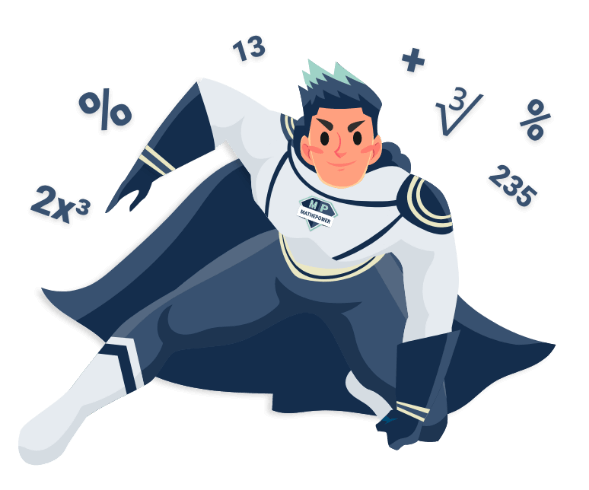# Transforming functionsTransforming functions Enter your function here. How shall your function be transformed? By in x-direction stretched shrinked By in y-direction stretched shrinked By to the the right l the left By to the up down

## How to transform the graph of a function?

This depends on the direction you want to transoform. In general, transformations in y-direction are easier than transformations in x-direction, see below.

## How to move a function in y-direction?

Just add the transformation you want to to. This is it. For example, letsmove this Graph byunits to the top.

The functionshall be moved by

5 to up

Graph before the transformation: :
 Dein Browser unterst�tzt den HTML-Canvas-Tag nicht. Hol dir einen neuen. :P
Function after the transformation:Dein Browser unterst�tzt den HTML-Canvas-Tag nicht. Hol dir einen neuen. :P
This is what Mathepower calculated:

Move the graph ofby 5 in direction up
Moved function:Simplify the new function: :| addto## How to move a function in x-direction?

If you want to move in x-direction, it is more difficult for two reasons:
• You have to replace every x by• and mind the sign: If you want to go in x-direction, replace x by. But if you want to go in the opposite direction, you replace x by.
Here is another example involving the latter function.

The functionshall be moved by

2 to the right

Graph before the transformation: :
 Dein Browser unterst�tzt den HTML-Canvas-Tag nicht. Hol dir einen neuen. :P
Function after the transformation:Dein Browser unterst�tzt den HTML-Canvas-Tag nicht. Hol dir einen neuen. :P
This is what Mathepower calculated:

Move the graph ofby 2 in direction right :
Replace every x byMoved function:Simplify the new function: :| Apply the higher binomial formulawith a=and b=| Calculateto the power of.| Expandand.| addto| addto| addto## How to stretch a function in y-direction?

This is easy, again: Just multiply your whole function by the stretching factor. For example, lets stretchby Factorin y-direction.

The functionshall be moved by

2 in y-direction stretched

Graph before the transformation: :
 Dein Browser unterst�tzt den HTML-Canvas-Tag nicht. Hol dir einen neuen. :P
Function after the transformation:Dein Browser unterst�tzt den HTML-Canvas-Tag nicht. Hol dir einen neuen. :P
This is what Mathepower calculated:

Transform the functionby 2 in y-direction stretch :
Multiply the function by 2
Stretched functionSimplify the new function: :| Expandand.## How to stretch a function in x-direction?

Again, like moving, stretchig is more difficult: We have to replace every x by(Mind that it is again not the way you may think: Stretching does not mean multiplying by, but dividing byThe functionshall be moved by

2 in x-direction stretched

Graph before the transformation: :
 Dein Browser unterst�tzt den HTML-Canvas-Tag nicht. Hol dir einen neuen. :P
Function after the transformation:Dein Browser unterst�tzt den HTML-Canvas-Tag nicht. Hol dir einen neuen. :P
This is what Mathepower calculated:

Transform the functionby 2 in x-direction stretch :
Replace every x byStretched functionSimplify the new function: :| Extractfrom the fraction| Solve with the power laws :equals| Extractfrom the fraction## And if I want to move another function?

This is Mathepower. Just enter it above.